## Weight initialization for neural networks

### Symmetrie breaking

The weights to different neurons of a full connected layer must be different initialized. If not all neurons in a layer have the same input and gradient. So they behave all equal.

Usually by random initialization of the weights the so called symmetrie breaking is achieved.

### Influence of the non-linear activations functions

To examine the influence of the non-linear activations functions $g$, note that the error responsibilities for the hidden units are given by (see here):

$$\vec \delta^{(l)} = g'(\vec z^{(l)}) \circ ( (\theta^{(l)})^T \cdot \vec \delta^{(l+1)} )$$

So if $z^{(l)}$ is small or large the $g'(\vec z^{(l)})$ are nearly zero for sigmoid shaped activation functions. The units are saturatated.

With small or nearly zero $\delta$'s the learning (weight changes) will be very slow for gradient descent because of the learning rule:

$$\theta^{(l)} \leftarrow \theta^{(l)} - \alpha (\vec a^{(l)} \times \vec \delta^{(l+1)})$$

To get a feeling about the range in which large derivatives can be expected, let's print the concrete values of $g'(z)$ for the common tanh and logistic activation functions:

In :
import numpy as np

def logistic_function(z):
return 1./(1 + np.exp(-z))

# d(logistic(x))/dx = logistic(x) * (1. - logistic(x))
def derivative_logistic_function(z):
return logistic_function(z) * (1. - logistic_function(z))

#d(tanh(x))/dx = 1 - tanh^2(x)
def derivative_tanh(z):
return 1. - np.tanh(z)**2

for z in np.linspace(-20.,20,20):
dt = derivative_tanh(z)
dl = derivative_logistic_function(z)
print "z:%f\t d(tanh(z))/dx: %e \t d(logistic(z))/dx: %e"%(z,dt, dl)

z:-20.000000	 d(tanh(z))/dx: 0.000000e+00 	 d(logistic(z))/dx: 2.061154e-09
z:-17.894737	 d(tanh(z))/dx: 1.110223e-15 	 d(logistic(z))/dx: 1.692055e-08
z:-15.789474	 d(tanh(z))/dx: 7.727152e-14 	 d(logistic(z))/dx: 1.389052e-07
z:-13.684211	 d(tanh(z))/dx: 5.201173e-12 	 d(logistic(z))/dx: 1.140307e-06
z:-11.578947	 d(tanh(z))/dx: 3.505209e-10 	 d(logistic(z))/dx: 9.360928e-06
z:-9.473684	 d(tanh(z))/dx: 2.362231e-08 	 d(logistic(z))/dx: 7.683595e-05
z:-7.368421	 d(tanh(z))/dx: 1.591954e-06 	 d(logistic(z))/dx: 6.300683e-04
z:-5.263158	 d(tanh(z))/dx: 1.072793e-04 	 d(logistic(z))/dx: 5.125696e-03
z:-3.157895	 d(tanh(z))/dx: 7.204086e-03 	 d(logistic(z))/dx: 3.911821e-02
z:-1.052632	 d(tanh(z))/dx: 3.871813e-01 	 d(logistic(z))/dx: 1.917840e-01
z:1.052632	 d(tanh(z))/dx: 3.871813e-01 	 d(logistic(z))/dx: 1.917840e-01
z:3.157895	 d(tanh(z))/dx: 7.204086e-03 	 d(logistic(z))/dx: 3.911821e-02
z:5.263158	 d(tanh(z))/dx: 1.072793e-04 	 d(logistic(z))/dx: 5.125696e-03
z:7.368421	 d(tanh(z))/dx: 1.591954e-06 	 d(logistic(z))/dx: 6.300683e-04
z:9.473684	 d(tanh(z))/dx: 2.362231e-08 	 d(logistic(z))/dx: 7.683595e-05
z:11.578947	 d(tanh(z))/dx: 3.505209e-10 	 d(logistic(z))/dx: 9.360928e-06
z:13.684211	 d(tanh(z))/dx: 5.201173e-12 	 d(logistic(z))/dx: 1.140307e-06
z:15.789474	 d(tanh(z))/dx: 7.727152e-14 	 d(logistic(z))/dx: 1.389052e-07
z:17.894737	 d(tanh(z))/dx: 1.110223e-15 	 d(logistic(z))/dx: 1.692055e-08
z:20.000000	 d(tanh(z))/dx: 0.000000e+00 	 d(logistic(z))/dx: 2.061154e-09



A graph says more than a bunch of numbers:

In :
%matplotlib inline
import matplotlib.pyplot as plt

z = np.linspace(-20.,20,200)
dt = derivative_tanh(z)
dl = derivative_logistic_function(z)
fig = plt.figure(figsize=(16, 5))
ax1.plot(z, dt, label="d(tanh)/dx")
ax1.plot(z, dl, label="d(logistic)/dx")
ax1.set_title("Derivatives of activation functions - linear y-axis")
ax1.set_xlabel("z")
ax1.set_ylabel("dg(z)/dz")
ax1.legend()
ax2.plot(z, dt, label="d(tanh)/dx")
ax2.plot(z, dl, label="d(logistic)/dx")
ax2.set_title("Derivatives of activation functions - logarithmic y-axis")
ax2.legend()
ax2.set_xlabel("z")
ax2.set_ylabel("log(dg(z)/dz)")
ax2.set_yscale('log')

plt.show()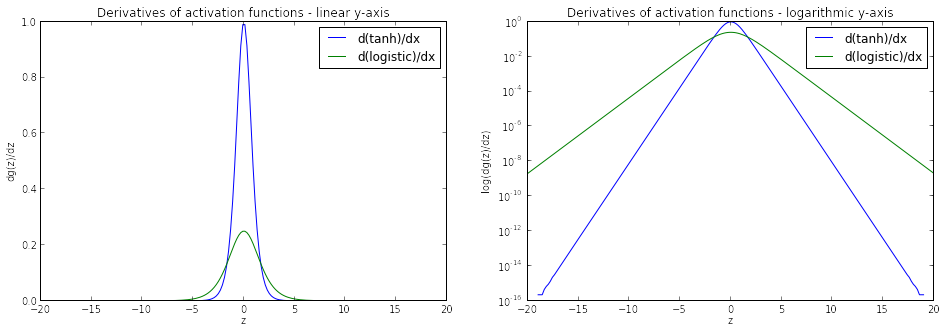For the logistic function and for random $z$ (symmetric around 0) the mean of the output of the logistic function is positive. In contrast the mean of the tanh is zero.

The $z$ values of the next layer are computed by $$z_i^{(l+1)} = \sum_{j=0}^{n} \theta_{ij}^{(l)} a_j^{(l)}$$

Note that the $\theta_{ij}$ should be positive or negative. So for the logistic function the expectation value of $z_i$ is $0$, also.

### Simulation for logistic function

A saturation of the units should be avoided but if we initialize the weights randomly with large values there is such a saturation. To demonstrate this, let's assume that about half of the neurons are active ($a = 1$) and the other are inactive ($a=0$).

This is similar to a one-dimesional random walk. So we expect a gaussian distribution of the probability density of the $z$-values with zero mean.

In :
def z_logistic(fan_in, sigma_w):
a = np.random.binomial(1, 0.5, size=fan_in)
#a = np.random.uniform(low=0, high=1., size=fan_in)

# initalize the weights randomly by a uniform distribution
w = np.random.uniform(-sigma_w, sigma_w, size=fan_in)# * 2. - 1.
return np.sum(a * w)

fan_in = 200

nb = 10000

#plt.hist(zs, bins=int(np.sqrt(nb)))
#plt.show()

def plot_pdensities(zs, g, derivative):
fig = plt.figure(figsize=(16, 10))
ax1.hist(zs, bins=int(np.sqrt(nb)), normed=True)
ax1.set_title("Probability density of z-values")
ax1.set_xlabel("z")
ax1.set_ylabel("p(z)")

ax1.hist(g(zs), bins=int(np.sqrt(nb)), normed=True)
ax1.set_title("Probability density of activity-values")
ax1.set_xlabel("a")
ax1.set_ylabel("p(a)")

ax2.hist(derivative(zs), bins=int(np.sqrt(nb)), normed=True)
ax2.set_title("Probability density of d(g(z))/dz")
ax2.set_xlabel("d(logistic(z))/dz")
ax2.set_ylabel("p(d(logistic(z))/dz)")

zs = np.ndarray(nb)
sigma_w = 1.

for i in range(nb):
zs[i] = z_logistic(fan_in, sigma_w)
plot_pdensities(zs, logistic_function, derivative_logistic_function)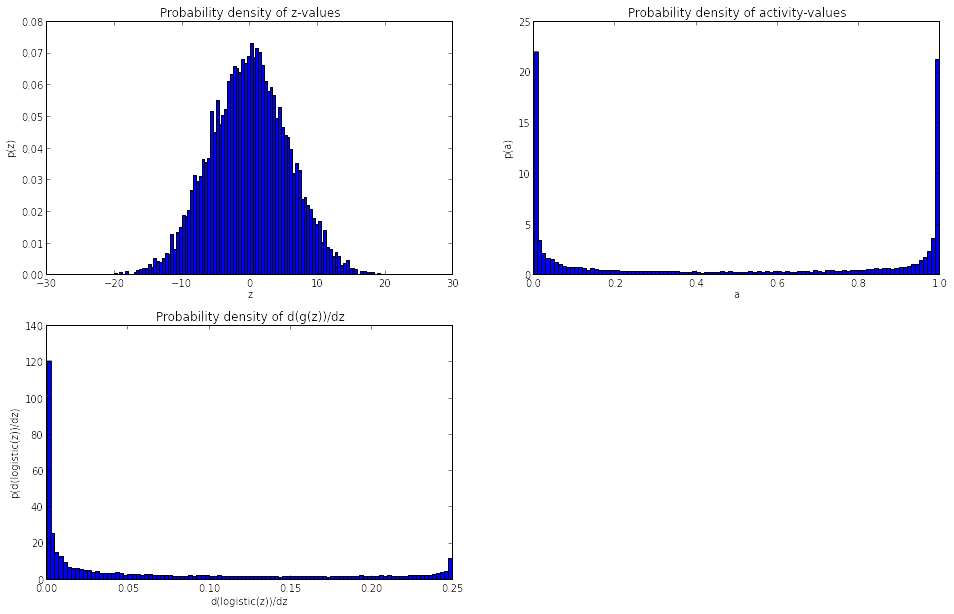Because of the large $\sigma$ of the gaussian probability density, many neurons are saturated. There are many neurons in the layer below and we have not adapted the range of the initialization uniform distribution to that number.

A common heuristic for weight initialization to prevent the saturation is [Glo10]

$$W_{ij} \sim U [ - \frac{1}{fan\_in}, \frac{1}{fan\_in}]$$

with

• $fan\_in$: the number of inputs to a neuron.
• $U$: Uniform distribution

This results in a much "nicer" distribution. So most of the neurons have no vanishing derivations.

In :
sigma_w = 1./np.sqrt(fan_in)
for i in range(nb):
zs[i] = z_logistic(fan_in, sigma_w)
plot_pdensities(zs, logistic_function, derivative_logistic_function)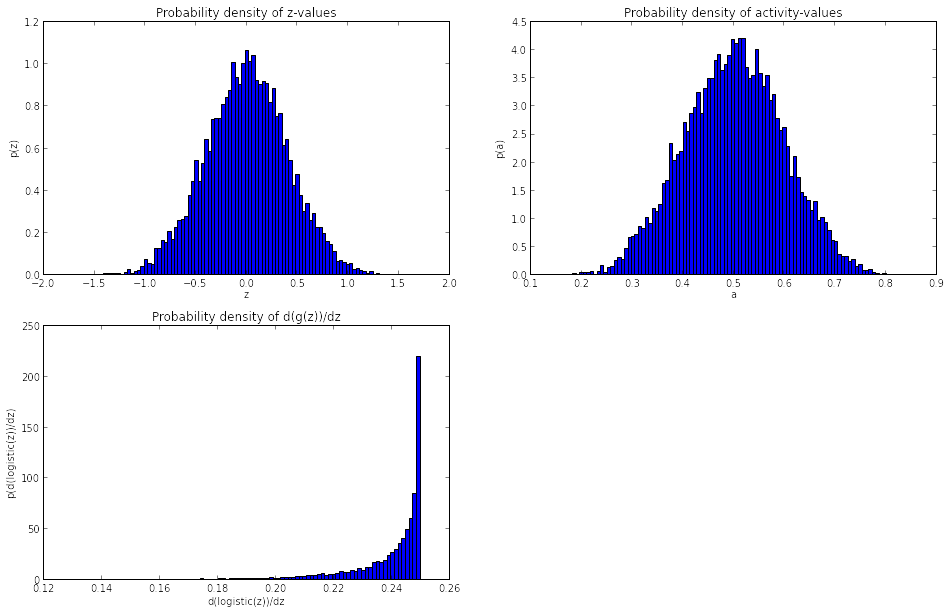### Tanh

The same argument holds for tanh units:

In :
def z_tanh(fan_in, sigma_w):
#a = np.random.binomial(1, 0.5, size=fan_in) * 2 - 1
a = np.random.uniform(-1., 1., size=fan_in)
w = np.random.uniform(-sigma_w, sigma_w, size=fan_in)
return np.sum(a * w)

sigma_w = 1.
for i in range(nb):
zs[i] = z_tanh(fan_in, sigma_w)
plot_pdensities(zs, np.tanh, derivative_tanh)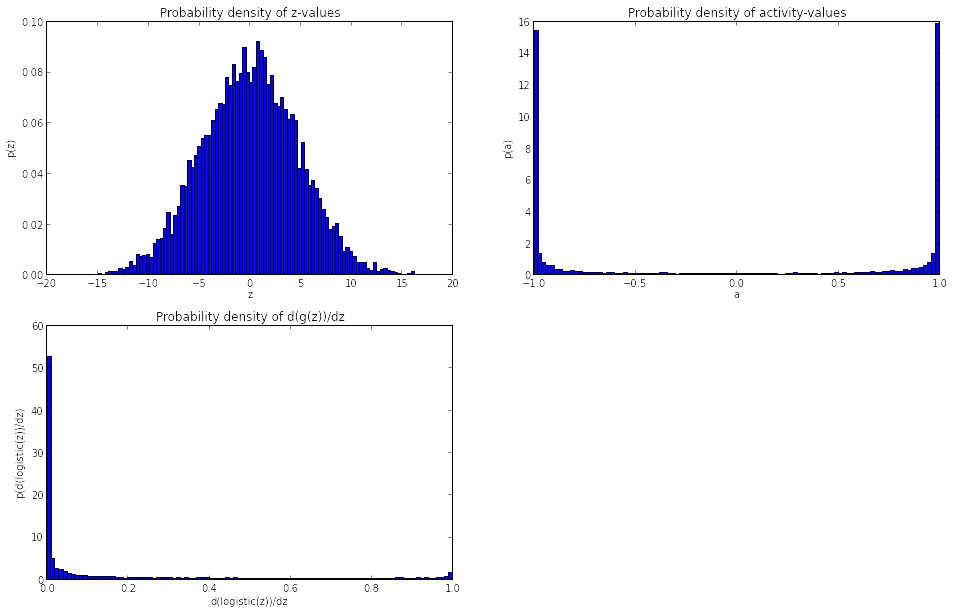In :
sigma_w = 1./np.sqrt(fan_in)
for i in range(nb):
zs[i] = z_tanh(fan_in, sigma_w)
plot_pdensities(zs, np.tanh, derivative_tanh)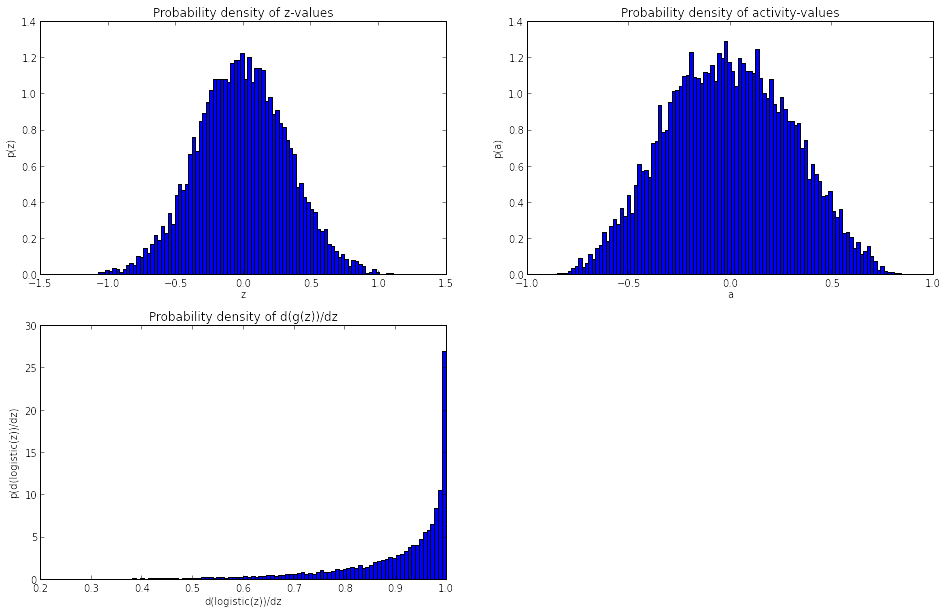#### Tanh vs. sigmoid units

"For hidden units the logistic sigmoid activation is unsuited" [Glo10] see also [Le98]

TODO: Why?? According to the derivation of the activation function the contrary should be the case.

#### Deep Feetworward Neural Networks

According to [Glo10] for deep networks the $fan\_out$ should be taken into consideration, too.

For tanh: $$W_{ij} \sim U [ - \frac{6}{fan\_in + fan\_out}, \frac{6}{fan\_in + fan\_out}]$$

For the logistic function: $$W_{ij} \sim U [ - \frac{4 * 6}{fan\_in + fan\_out}, \frac{4 * 6}{fan\_in + fan\_out}]$$

In :
import theano
def init_W_b(W, b, n_in, n_out):

if W is None:
W_values = numpy.asarray(
rng.uniform(
low=-np.sqrt(6./(n_in + n_out)),
high=np.sqrt(6./(n_in + n_out)),
size=(n_in, n_out)
),
dtype=theano.config.floatX
)
W = theano.shared(value=W_values, name='W', borrow=True)

# init biases to appropriate values depending on the activation functions
#
#
if b is None:
b_values = numpy.ones((n_out,), dtype=theano.config.floatX) * np.cast[theano.config.floatX](0.01)
b = theano.shared(value=b_values, name='b', borrow=True)
return W, b


## Sparse Initialization

Martens [Mar10] proposes another initialization scheme: "limit the number of non-zero incoming connection weights to each unit".

In :
import random
def init_W_b(W, b, n_in, n_out, b_init=0., nb_sparse=15):

if W is None:
W_values = np.zeros(shape=(n_in, n_out))
nb_sparse = min(n_in, nb_sparse)
for i in xrange(n_out):
non_zero_indexes = random.sample(xrange(n_in), nb_sparse)
W_values[non_zero_indexes, i] = np.random.normal(size=(len(non_zero_indexes),))
W = theano.shared(value=W_values, name='W', borrow=True)

# init biases to appropriate values depending on the activation functions
# 0 or
# 0.5 for tanh units
if b is None:
b_values = np.zeros((n_out,), dtype=theano.config.floatX) + np.cast[theano.config.floatX](b_init)
b = theano.shared(value=b_values, name='b', borrow=True)
return W, b

W, b = init_W_b(None, None, n_in=40, n_out=5)